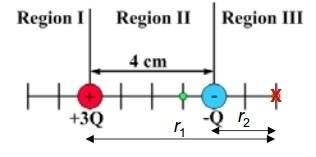# Places electric potential is zero when 2 charges

• JustStudying
In summary, the electric potential is zero at the red cross in region III due to the cancelation of the potentials from the charges in that region. Additionally, the electric potential is zero at the green dot in region II due to the same cancelation of potentials.f

## Homework Statementi'm having a bit of trouble understanding/visualising why the electric potential is zero in the red cross within region III and the green dot within region II

from my current understanding, the green dot is where the potentials from both charges are equal and opposite therefore cancel out (am I right?), but I have no idea why the electric potential is zero at the red cross

can anyone help me out?

V = kq/r

## The Attempt at a Solution

Calculate the voltages separately for each of the two charges, for each of the positions along the line. Note that the voltages decrease as the point is further away.

Then see which ones add up to zero at the same point.

Note that if the two charges were equal, then the zero point would be midway between them.

The total Electric potential due to these two charges at the red cross must be summed as scalars. So write down the potentials as a sum to find the total potentials that's it.

I was hoping if anyone could explain why there are TWO points where the potential is zero i.e I simply don't get why the potential is zero at the red cross - the 3q charge is not even in region III so how could it cause a zero potential there?, the actual number crunching isn't too difficult.

Generate a plot. Vary the positions and charges.

Remember that the potential goes off in both directions, as far as you can go.

I was hoping if anyone could explain why there are TWO points where the potential is zero i.e I simply don't get why the potential is zero at the red cross - the 3q charge is not even in region III so how could it cause a zero potential there?, the actual number crunching isn't too difficult.
The regions are arbitrary definitions on your line. Both charges influence the potential everywhere.

If you extend the analysis to two dimensions, you get a full circle of points with zero potential.

Please do not open multiple threads for the same topic, I deleted the other one.

•1 person